# High School Chemistry : Using Isotopic Notation

## Example Questions

### Example Question #1 : Using Isotopic Notation

An atom of mercury can be written as follows: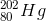What is the atomic number for mercury?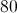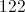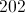Explanation:

The atomic number is the number of protons present in the nucleus of an atom. This number does not change without changing the element. In elemental notation, the atomic number is found at the bottom left corner of the chemical symbol for the element. The upper number represents the nuclear mass of the atom, given by the sum of the protons and neutrons.

### Example Question #1 : Nuclear Chemistry

Consider the following ion: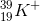How many neutrons are in the ion?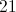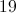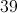Explanation:

Elemental notation gives us insight into the particles that are found in a given atom. The atomic number in the lower left corner shows how many protons are in an atom, and the mass number in the upper left corner shows how many protons and neutrons are in an atom. As a result, we can find the number of neutrons in the ion in question by subtracting the atomic number from the mass number: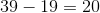There are twenty neutrons in this potassium ion.

### Example Question #3 : Nuclear Chemistry

A cobalt atom of atomic mass 60 undergoes an alpha decay, two beta decays, and another alpha decay. What is the identity of the daughter nuclide?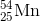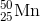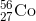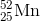Explanation:

Let's start by identifying our starting atom. Cobalt has 27 protons. If the given atom has an atomic mass of 60, then it must have 33 neutrons.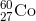An alpha decay is the emission of a helium nucleus with mass 4 (2 protons and 2 neutrons). This results in a daughter nuclide whose atomic number is 2 less and atomic mass is 4 less than the original element.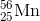A beta decay is the conversion of a neutron to a proton, accompanied by the emission of an electron. This results in a daughter nuclide whose atomic number is 1 more than the original element, with no change to atomic mass. This process is done twice.Doing 1 alpha and 2 beta decays gives a net change of -4 for the atomic mass and 0 for the atomic number.

### Example Question #2 : Using Isotopic Notation

Identify the unknown element that is formed in the following nuclear reaction: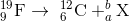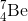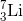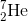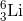Explanation:

To do this problem, all you have to remember is that the sum of the atomic numbers and atomic masses should be equal on the left and right sides.

Mass values: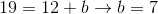Atomic numbers: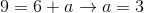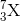Look at your periodic table to find the identity of the resulting element.### Example Question #1 : Nuclear Chemistry

The following reaction shows what type of decay?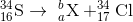Gamma decay

Positron emission

Alpha decay

Beta decay

Beta decay

Explanation:

The resulting element has the same atomic mass and the atomic number has increased by 1. This means that a neutron has been converted into a proton, and an electron has been emmitted; this happens in beta decay.

Beta decay: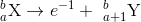### Example Question #6 : Nuclear Chemistry

How many neutrons in the following isotope contain?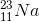Explanation: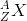This is the standard isotopic notation.is the mass number which is equal to the number of protons plus neutrons, and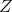is equal to the atomic number. Thus,is a sodium atom with 12 neutrons.

### Example Question #1 : Nuclear Chemistry

How many neutrons does the follwing isotope have?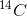Explanation:This is the standard isotopic notation.is the mass number which is equal to the number of protons plus neutrons, andis equal to the atomic number. Sometimes, the atomic number is left out since it can be easily found using the atomic symbol and the periodic table. Thus,is a carbon atom (all of which have 6 protons) with 8 neutrons, giving us a mass number of 14.

### Example Question #1 : Using Isotopic Notation

Which of the following is not true about chemical isotopes?

Isotopes can have different number of neutrons

All of these

Isotopes can have different chemical properties

Isotopes can have different number of protons

Isotopes can have different atomic weights

Isotopes can have different number of protons

Explanation:

Isotopes of elements have different numbers of neutrons, and different atomic weights, but must have the same number of protons. For example, carbon exists as isotopes of C12, C13, and C14, but these are all carbon atoms and have exactly 6 protons. The number of neutrons, and thus atomic weight varies between isotopes. Also, different isotopes may have different chemical properties, such as half-life and type of radioactive decay.

### Example Question #2 : Using Isotopic Notation

An isotope can be written in isotopic notation. If an isotope is written as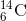identify the isotope in the format of "element-atomic mass number" as well as identify how many neutrons the isotope has.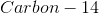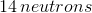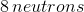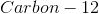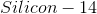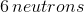Explanation:

The element is either found by looking at the symbol "C" and identifying it on the periodic table as carbon, or by looking at the atomic number. The isotopic notation given is in the form of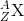Where X is the symbol for the element, Z is the atomic number (number of protons) and A is the atomic mass number (number of protons plus number of neutrons).

Since the atomic number is 6 we can also find this on the periodic table to be carbon. The isotope now can be written asTo find the number of neutrons we take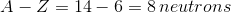### All High School Chemistry Resources